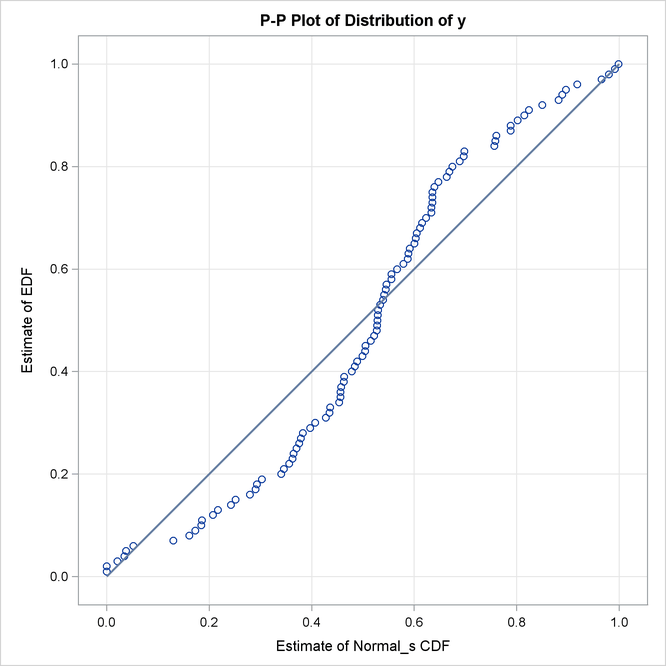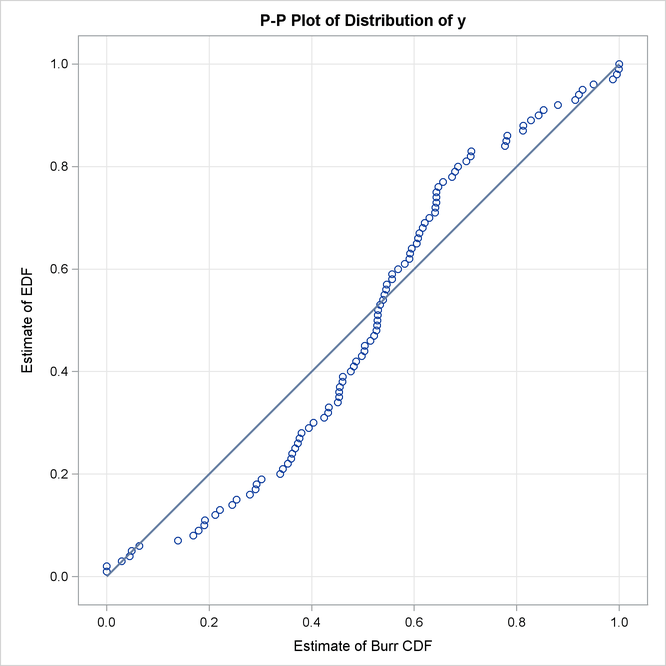# The SEVERITY Procedure

### Example 23.2 Defining a Model for the Gaussian Distribution with a Scale Parameter

If you want to estimate the influence of regression effects, then the model needs to be parameterized to have a scale parameter. Although this might not be always possible, it is possible for the Gaussian distribution by replacing the location parameterwith another parameter,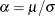, and defining the PDF (f) and the CDF (F) as follows: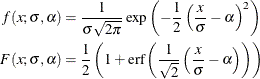You can verify thatis the scale parameter, because both of the following equalities are true: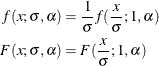Note: The Gaussian distribution is not a commonly used severity distribution. It is used in this example primarily to illustrate the concept of parameterizing a distribution such that it has a scale parameter. Although the distribution has a support over the entire real line, you can fit the distribution with PROC SEVERITY only if the input sample contains nonnegative values.

The following statements use the alternate parameterization to define a new model named NORMAL_S. The definition is stored in the Work.Sevexmpl library.

/*-------- Define Normal Distribution With Scale Parameter  ----------*/
proc fcmp library=sashelp.svrtdist outlib=work.sevexmpl.models;
function normal_s_pdf(x, Sigma, Alpha);
/* Sigma : Scale & Standard Deviation */
/* Alpha : Scaled mean */
return ( exp(-(x/Sigma - Alpha)**2/2) /
(Sigma * sqrt(2*constant('PI'))) );
endsub;

function normal_s_cdf(x, Sigma, Alpha);
/* Sigma : Scale & Standard Deviation */
/* Alpha : Scaled mean */
z = x/Sigma - Alpha;
return (0.5 + 0.5*erf(z/sqrt(2)));
endsub;

subroutine normal_s_parminit(dim, x[*], nx[*], F[*], Ftype, Sigma, Alpha);
outargs Sigma, Alpha;
array m / nosymbols;

/* Compute estimates by using method of moments */
call svrtutil_rawmoments(dim, x, nx, 2, m);
Sigma = sqrt(m - m**2);
Alpha = m/Sigma;
endsub;

subroutine normal_s_lowerbounds(Sigma, Alpha);
outargs Sigma, Alpha;
Alpha = .; /* Alpha has no lower bound */
Sigma = 0; /* Sigma > 0 */
endsub;
quit;



An important point to note is that the scale parameter Sigma is the first distribution parameter (after the 'x' argument) listed in the signatures of NORMAL_S_PDF and NORMAL_S_CDF functions. Sigma is also the first distribution parameter listed in the signatures of other subroutines. This is required by PROC SEVERITY, so that it can identify which is the scale parameter. When you specify regression effects, PROC SEVERITY checks whether the first parameter of each candidate distribution is a scale parameter (or a log-transformed scale parameter if dist_SCALETRANSFORM subroutine is defined for the distribution with LOG as the transform). If it is not, then an appropriate message is written the SAS log and that distribution is not fitted.

Let the following DATA step statements simulate a sample from the normal distribution where the parameteris affected by the regressors as follows: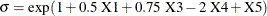The sample is simulated such that the regressor X2 is linearly dependent on regressors X1 and X3.

/*--- Simulate a Normal sample affected by Regressors ---*/
data testnorm_reg(keep=y x1-x5 Sigma);
array x{*} x1-x5;
array b{6} _TEMPORARY_ (1 0.5 . 0.75 -2 1);
call streaminit(34567);
label y='Normal Response Influenced by Regressors';

do n = 1 to 100;
/* simulate regressors */
do i = 1 to dim(x);
x(i) = rand('UNIFORM');
end;
/* make x2 linearly dependent on x1 and x3 */
x(2) = x(1) + 5 * x(3);

/* compute log of the scale parameter */
logSigma = b(1);
do i = 1 to dim(x);
if (i ne 2) then
logSigma = logSigma + b(i+1) * x(i);
end;

Sigma = exp(logSigma);
y = rand('NORMAL', 25, Sigma);
output;
end;
run;


The following statements use PROC SEVERITY to fit the NORMAL_S distribution model along with some of the predefined distributions to the simulated sample:

/*--- Set the search path for functions defined with PROC FCMP ---*/
options cmplib=(work.sevexmpl);

/*-------- Fit models with PROC SEVERITY --------*/
proc severity data=testnorm_reg print=all plots=none;
loss y;
scalemodel x1-x5;
dist Normal_s burr logn pareto weibull;
run;



The "Model Selection" table in Output 23.2.1 indicates that all the models, except the Burr distribution model, have converged. Also, only three models, Normal_s, Burr, and Weibull, seem to have a good fit for the data. The table that compares all the fit statistics indicates that Normal_s model is the best according to the likelihood-based statistics; however, the Burr model is the best according to the EDF-based statistics.

Output 23.2.1: Summary of Results for Fitting the Normal Distribution with Regressors

The SEVERITY Procedure

Input Data Set
Name WORK.TESTNORM_REG

Model Selection
Distribution Converged -2 Log
Likelihood
Selected
Normal_s Yes 603.95786 Yes
Burr Maybe 612.81685 No
Logn Yes 749.20125 No
Pareto Yes 841.07022 No
Weibull Yes 612.77496 No

All Fit Statistics
Distribution -2 Log
Likelihood
AIC AICC BIC KS AD CvM
Normal_s 603.95786 * 615.95786 * 616.86108 * 631.58888 * 1.52388   4.00152   0.70769
Burr 612.81685   626.81685   628.03424   645.05304   1.50448 * 3.90072 * 0.63399 *
Logn 749.20125   761.20125   762.10448   776.83227   2.88110   16.20558   3.04825
Pareto 841.07022   853.07022   853.97345   868.70124   4.83810   31.60568   6.84046
Weibull 612.77496   624.77496   625.67819   640.40598   1.50490   3.90559   0.63458

This prompts you to further evaluate why the model with Burr distribution has not converged. The initial values, convergence status, and the optimization summary for the Burr distribution are shown in Output 23.2.2. The initial values table indicates that the regressor X2 is redundant, which is expected. More importantly, the convergence status indicates that it requires more than 50 iterations. PROC SEVERITY enables you to change several settings of the optimizer by using the NLOPTIONS statement. In this case, you can increase the limit of 50 on the iterations, change the convergence criterion, or change the technique to something other than the default trust-region technique.

Output 23.2.2: Details of the Fitted Burr Distribution Model

The SEVERITY Procedure
Burr Distribution

Distribution Information
Name Burr
Description Burr Distribution
Distribution Parameters 3
Regression Parameters 4

Initial Parameter Values and Bounds
Parameter Initial
Value
Lower
Bound
Upper
Bound
Theta 25.75198 1.05367E-8 Infty
Alpha 2.00000 1.05367E-8 Infty
Gamma 2.00000 1.05367E-8 Infty
x1 0.07345 -709.78271 709.78271
x2 Redundant
x3 -0.14056 -709.78271 709.78271
x4 0.27064 -709.78271 709.78271
x5 -0.23230 -709.78271 709.78271

Convergence Status
Needs more than 50 iterations.

Optimization Summary
Optimization Technique Trust Region
Iterations 50
Function Calls 137
Log Likelihood -306.40842

The following PROC SEVERITY step uses the NLOPTIONS statement to change the convergence criterion and the limits on the iterations and function evaluations, exclude the lognormal and Pareto distributions that have been confirmed previously to fit the data poorly, and exclude the redundant regressor X2 from the model:

/*--- Refit and compare models with higher limit on iterations ---*/
proc severity data=testnorm_reg print=all plots=pp;
loss y;
scalemodel x1 x3-x5;
dist Normal_s burr weibull;
nloptions absfconv=2.0e-5 maxiter=100 maxfunc=500;
run;



The results shown in Output 23.2.3 indicate that the Burr distribution has now converged and that the Burr and Weibull distributions have an almost identical fit for the data. The NORMAL_S distribution is still the best distribution according to the likelihood-based criteria.

Output 23.2.3: Summary of Results after Changing Maximum Number of Iterations

The SEVERITY Procedure

Input Data Set
Name WORK.TESTNORM_REG

Model Selection
Distribution Converged -2 Log
Likelihood
Selected
Normal_s Yes 603.95786 Yes
Burr Yes 612.79276 No
Weibull Yes 612.77496 No

All Fit Statistics
Distribution -2 Log
Likelihood
AIC AICC BIC KS AD CvM
Normal_s 603.95786 * 615.95786 * 616.86108 * 631.58888 * 1.52388   4.00152   0.70769
Burr 612.79276   626.79276   628.01015   645.02895   1.50472 * 3.90351 * 0.63433 *
Weibull 612.77496   624.77496   625.67819   640.40598   1.50490   3.90559   0.63458

The comparison of the PDF estimates of all the candidates is shown in Output 23.2.4. Each plotted PDF estimate is an average computed over the N PDF estimates that are obtained with the scale parameter determined by each of the N observations in the input data set. The PDF plot shows that the Burr and Weibull models result in almost identical estimates. All the estimates have a slight left skew with the mode closer to Y=25, which is the mean of the simulated sample.

Output 23.2.4: Comparison of EDF and CDF Estimates of the Fitted Models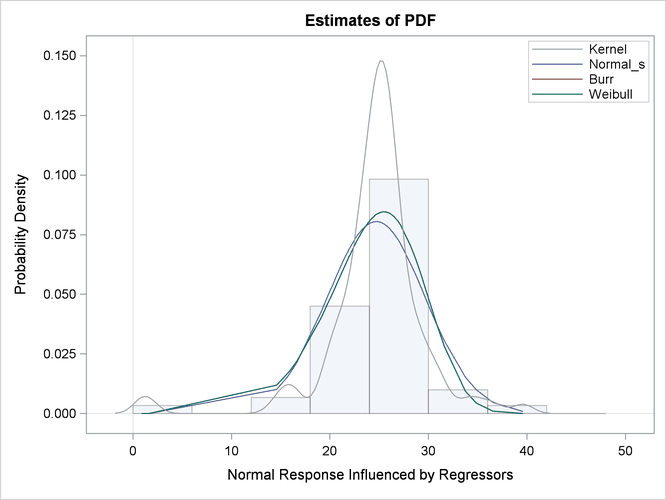The P-P plots for the Normal_s and Burr distributions are shown in Output 23.2.5. These plots show how the EDF estimates compare against the CDF estimates. Each plotted CDF estimate is an average computed over the N CDF estimates that are obtained with the scale parameter determined by each of the N observations in the input data set. Comparing the P-P plots of Normal_s and Burr distributions indicates that both fit the data almost similarly, but the Burr distribution fits the right tail slightly better, which explains why the EDF-based statistics prefer it over the Normal_s distribution.

Output 23.2.5: Comparison of EDF and CDF Estimates of NORMAL_S and BURR Models